Courses
Courses for Kids
Free study material
Offline Centres
More

# NCERT Solutions for Class 10 Maths Chapter 8 - ExerciseLast updated date: 23rd Nov 2023
Total views: 529.8k
Views today: 11.29k

## NCERT Solutions for Class 10 Maths Chapter 8 Introduction to Trigonometry (Ex 8.1) Exercise 8.1

NCERT Solutions for Class 10 Maths Chapter 8, Introduction to Trigonometry, Exercise 8.1, is available here in PDF format. The solutions can be downloaded easily and can be referred by the students from anywhere. The NCERT solutions created by our subject experts are explained in a step-by-step procedure along with the related geometric figure. The solutions are prepared per the latest NCERT syllabus and guidelines of the CBSE board and it aims to help the students to excel in their board and competitive examination.

## Class 10 Maths Chapter 8 Exercise 8.1: Topics Covered

• Introduction To Trigonometry

• Trigonometric Identities

• Trigonometry Ratios

### What is Trigonometry?

Trigonometry is the branch of mathematics that is related to the specific functions of angles and their application to calculations. There are six functions of an angle that are widely used in trigonometry to solve unknown sides. Their six functions of trigonometry and their abbreviations are sine (sin), cosine (cos), tangent (tan), cotangent (cot), secant (sec), and cosecant (cosec).

### What Are Trigonometric Identities?

Trigonometric Identities are termed as the equalities that include trigonometry functions and hold valid for all the values of variables given in the equation.

### What Are Trigonometry Ratios?

Trigonometry is a concept that represents the relation between the angles and sides of a right-angled triangle. As we know, there are three sides of a triangle named Hypotenuse, Adjacent, and Opposite. The ratio between these three sides of a triangle based on the angle between them is termed Trigonometric Ratios.

Exercise 8.1 Class 10 Maths NCERT solutions are the ultimate guide to learn the foundational concepts of trigonometry. Professionals at Vedantu provide well-constructed NCERT Solutions material for the well being of the students. Keeping the pressure the students undergo in mind, and the notes are simplified to their crisp form, making the learning process easier and comfortable.

Students can also download NCERT Solutions for Class 10 Science created by Expert Teachers at Vedantu for your ease.

Watch videos on
NCERT Solutions for Class 10 Maths Chapter 8 - ExerciseTRIGONOMETRY Class 10 in One Shot (Complete Chapter) | CBSE 10 Math Chapter 8 [Term 1 Exam] Vedantu
Vedantu 9&10
SubscribeShare
7.3K likes
153.7K Views
2 years agoTRIGONOMETRY L-2 (Trigonometric Identities) CBSE Class 10 Maths Chapter 8 | Term 1 Exam | Vedantu
Vedantu 9&10
8K likes
138.9K Views
2 years ago
Play QuizTRIGONOMETRY L-1 [Introduction and Trigonometric Ratios of Some Specific Angles] CBSE 10 Math Chap 8
Vedantu 9&10
13.5K likes
262.5K Views
2 years ago
Play QuizTrigonometry in One Shot | CBSE Class 10 Maths Chapter 8 | NCERT Solutions | Vedantu Class 9 and 10
Vedantu 9&10
7.7K likes
182.7K Views
3 years ago
See More## Access NCERT Solutions for Class -10 Maths Chapter 8 – Introduction to Trigonometry

Exercise 8.1

1. In $\Delta ABC$ right angled at $B$, $AB=24\text{ cm}$, $BC=7\text{ cm}$. Determine

i) $\sin A,\cos A$

Ans: Given that in the right angle triangle $\Delta ABC$, $AB=24\text{ cm}$, $BC=7\text{ cm}$.

Let us draw a right triangle $\Delta ABC$, also $AB=24\text{ cm}$, $BC=7\text{ cm}$. We get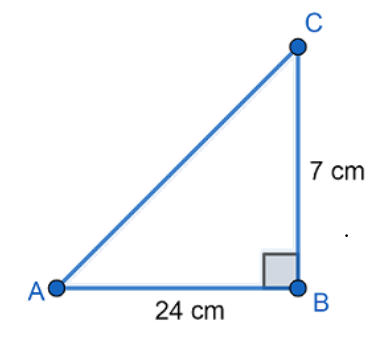We have to find $\sin A,\cos A$.

We know that for right triangle

$\sin \theta =\dfrac{\text{opposite side}}{\text{hypotenuse}}$ and

$\cos \theta =\dfrac{\text{adjacent side}}{\text{hypotenuse}}$

Now, we need to apply the Pythagoras theorem to find the measure of hypotenuse.

In $\Delta ABC$, by Pythagoras theorem ,

${{\left( \text{hypotenuse} \right)}^{2}}={{\left( base \right)}^{2}}+{{\left( perpendicular \right)}^{2}}$

Here, $AB=24\text{ cm}$, $BC=7\text{ cm}$

We get

$\Rightarrow {{\left( \text{AC} \right)}^{2}}={{\left( AB \right)}^{2}}+{{\left( BC \right)}^{2}}$

$\Rightarrow {{\left( \text{AC} \right)}^{2}}={{\left( 24 \right)}^{2}}+{{\left( 7 \right)}^{2}}$

$\Rightarrow {{\left( \text{AC} \right)}^{2}}=576+49$

$\Rightarrow {{\left( \text{AC} \right)}^{2}}=625\text{ }c{{m}^{2}}$

$\Rightarrow AC=25\text{ cm}$

Now,

$\sin \theta =\dfrac{\text{opposite side}}{\text{hypotenuse}}$

$\Rightarrow \sin A=\dfrac{BC}{AC}$

$\therefore \sin A=\dfrac{7}{25}$

$\cos \theta =\dfrac{\text{adjacent side}}{\text{hypotenuse}}$

$\Rightarrow \cos A=\dfrac{AB}{AC}$

$\therefore \cos A=\dfrac{24}{25}$

ii) $\sin C,\cos C$

Ans: Given that in the right angle triangle $\Delta ABC$, $AB=24\text{ cm}$, $BC=7\text{ cm}$.

Let us draw a right triangle $\Delta ABC$, also $AB=24\text{ cm}$, $BC=7\text{ cm}$. We getWe have to find $\sin C,\cos C$.

We know that for right triangle

$\sin \theta =\dfrac{\text{opposite side}}{\text{hypotenuse}}$ and

$\cos \theta =\dfrac{\text{adjacent side}}{\text{hypotenuse}}$

Now, we need to apply the Pythagoras theorem to find the measure of hypotenuse.

In $\Delta ABC$, by Pythagoras theorem ,

${{\left( \text{hypotenuse} \right)}^{2}}={{\left( base \right)}^{2}}+{{\left( perpendicular \right)}^{2}}$

Here, $AB=24\text{ cm}$, $BC=7\text{ cm}$

We get

$\Rightarrow {{\left( \text{AC} \right)}^{2}}={{\left( AB \right)}^{2}}+{{\left( BC \right)}^{2}}$

$\Rightarrow {{\left( \text{AC} \right)}^{2}}={{\left( 24 \right)}^{2}}+{{\left( 7 \right)}^{2}}$

$\Rightarrow {{\left( \text{AC} \right)}^{2}}=576+49$

$\Rightarrow {{\left( \text{AC} \right)}^{2}}=625\text{ }c{{m}^{2}}$

$\Rightarrow AC=25\text{ cm}$

Now,

$\sin \theta =\dfrac{\text{opposite side}}{\text{hypotenuse}}$

$\Rightarrow \sin C=\dfrac{AB}{AC}$

$\therefore \sin C=\dfrac{24}{25}$

$\cos \theta =\dfrac{\text{adjacent side}}{\text{hypotenuse}}$

$\Rightarrow \cos C=\dfrac{BC}{AC}$

$\therefore \cos A=\dfrac{7}{25}$

2. In the given figure find $\tan P-\cot R$.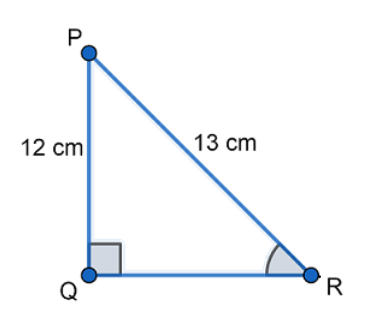Ans: Given in the figure,

$PQ=12\text{ cm}$

$PQ=13\text{ cm}$

We know that for right triangle

$\tan \theta =\dfrac{\text{opposite side}}{\text{adjacent side}}$ and

$\cot \theta =\dfrac{\text{adjacent side}}{\text{opposite side}}$

Now, we need to apply the Pythagoras theorem to find the measure of adjacent side/base.

In $\Delta PQR$, by Pythagoras theorem ,

${{\left( \text{hypotenuse} \right)}^{2}}={{\left( base \right)}^{2}}+{{\left( perpendicular \right)}^{2}}$

We get

$\Rightarrow {{\left( PR \right)}^{2}}={{\left( PQ \right)}^{2}}+{{\left( QR \right)}^{2}}$

$\Rightarrow {{\left( 13 \right)}^{2}}={{\left( 12 \right)}^{2}}+{{\left( QR \right)}^{2}}$

$\Rightarrow 169=144+{{\left( QR \right)}^{2}}$

$\Rightarrow {{\left( QR \right)}^{2}}=169-144$

$\Rightarrow {{\left( QR \right)}^{2}}=25\text{ }c{{m}^{2}}$

$\Rightarrow QR=5\text{ cm}$

Now,

$\tan P=\dfrac{\text{opposite side}}{\text{adjacent side}}$

$\Rightarrow \tan P=\dfrac{QR}{PQ}$

$\therefore \tan P=\dfrac{5}{12}$

$\cot R=\dfrac{\text{adjacent side}}{\text{opposite side}}$

$\Rightarrow \cot R=\dfrac{QR}{PQ}$

$\therefore \cot R=\dfrac{5}{12}$

$\Rightarrow \tan P-\cot R=\dfrac{5}{12}-\dfrac{5}{12}$

$\therefore \tan P-\cot R=0$

3. If $\sin A=\dfrac{3}{4}$, calculate $\cos A$ and $\tan A$.

Ans: Let us consider a right angled triangle $\Delta ABC$. We get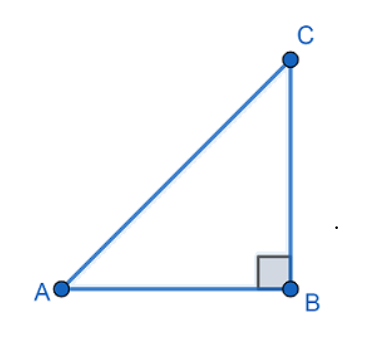Given that $\sin A=\dfrac{3}{4}$.

We know that  $\sin \theta =\dfrac{\text{opposite side}}{\text{hypotenuse}}$.

From the above figure, we get

$\sin A=\dfrac{BC}{AC}$

Therefore, we get

$\Rightarrow BC=3$ and

$\Rightarrow AC=4$

Now, we have to find the values of $\cos A$ and $\tan A$.

We know that $\cos \theta =\dfrac{\text{adjacent side}}{\text{hypotenuse}}$ and $\tan \theta =\dfrac{\text{opposite side}}{\text{adjacent side}}$.

Now, we need to apply the Pythagoras theorem to find the measure of adjacent side/base.

In $\Delta ABC$, by Pythagoras theorem ,

${{\left( \text{hypotenuse} \right)}^{2}}={{\left( base \right)}^{2}}+{{\left( perpendicular \right)}^{2}}$

Here, $AC=4\text{ cm}$, $BC=3\text{ cm}$

We get

$\Rightarrow {{\left( \text{AC} \right)}^{2}}={{\left( AB \right)}^{2}}+{{\left( BC \right)}^{2}}$

$\Rightarrow {{4}^{2}}=A{{B}^{2}}+{{3}^{2}}$

$\Rightarrow 16=A{{B}^{2}}+9$

$\Rightarrow A{{B}^{2}}=16-9$

$\Rightarrow A{{B}^{2}}=7$

$\Rightarrow AB=\sqrt{7}\text{ cm}$

Now, we get

$\cos A=\dfrac{AB}{AC}$

$\therefore \cos A=\dfrac{\sqrt{7}}{4}$

And $\tan A=\dfrac{BC}{AB}$

$\therefore \tan A=\dfrac{3}{\sqrt{7}}$

4. Given $15\cot A=8$. Find $\sin A$ and $\sec A$.

Ans: Let us consider a right angled triangle $\Delta ABC$. We getGiven that $15\cot A=8$.

We get $\cot A=\dfrac{8}{15}$.

We know that $\cot \theta =\dfrac{\text{adjacent side}}{\text{opposite side}}$.

From the above figure, we get

$\cot A=\dfrac{AB}{BC}$

Therefore, we get

$\Rightarrow BC=15$ and

$\Rightarrow AB=8$

Now, we have to find the values of $\sin A$ and $\sec A$.

We know that $\sin \theta =\dfrac{\text{opposite side}}{\text{hypotenuse}}$ and $\sec \theta =\dfrac{\text{hypotenuse}}{\text{adjacent side}}$.

Now, we need to apply the Pythagoras theorem to find the measure of hypotenuse.

In $\Delta ABC$, by Pythagoras theorem ,

${{\left( \text{hypotenuse} \right)}^{2}}={{\left( base \right)}^{2}}+{{\left( perpendicular \right)}^{2}}$

We get

$\Rightarrow {{\left( \text{AC} \right)}^{2}}={{\left( AB \right)}^{2}}+{{\left( BC \right)}^{2}}$

$\Rightarrow A{{C}^{2}}={{8}^{2}}+{{15}^{2}}$

$\Rightarrow A{{C}^{2}}=64+225$

$\Rightarrow A{{C}^{2}}=289$

$\Rightarrow AC=17\text{ cm}$

Now, we get

$\sin A=\dfrac{BC}{AC}$

$\therefore \sin A=\dfrac{15}{17}$

And $\sec A=\dfrac{AC}{AB}$

$\therefore \sec A=\dfrac{17}{8}$

5. Given $\sec \theta =\dfrac{13}{12}$, calculate all other trigonometric ratios.

Ans: Let us consider a right angled triangle $\Delta ABC$. We get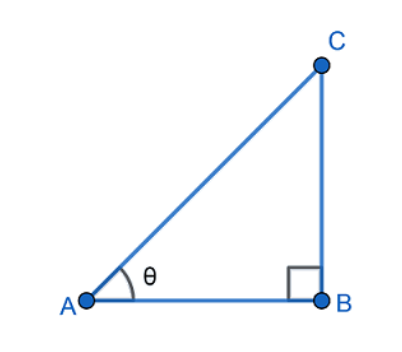Given that $\sec \theta =\dfrac{13}{12}$.

We know that $\sec \theta =\dfrac{\text{hypotenuse}}{\text{adjacent side}}$.

From the above figure, we get

$\sec \theta =\dfrac{AC}{AB}$

Therefore, we get

$\Rightarrow AC=13$ and

$\Rightarrow AB=12$

Now, we need to apply the Pythagoras theorem to find the measure of the perpendicular/opposite side.

In $\Delta ABC$, by Pythagoras theorem ,

${{\left( \text{hypotenuse} \right)}^{2}}={{\left( base \right)}^{2}}+{{\left( perpendicular \right)}^{2}}$

We get

$\Rightarrow {{\left( \text{AC} \right)}^{2}}={{\left( AB \right)}^{2}}+{{\left( BC \right)}^{2}}$

$\Rightarrow {{13}^{2}}={{12}^{2}}+B{{C}^{2}}$

$\Rightarrow 169=144+B{{C}^{2}}$

$\Rightarrow B{{C}^{2}}=25$

$\Rightarrow BC=5\text{ cm}$

Now, we know that

$\sin \theta =\dfrac{\text{opposite side}}{\text{hypotenuse}}$

Here, $\sin \theta =\dfrac{BC}{AC}$

$\therefore \sin \theta =\dfrac{5}{13}$

We know that $\cos \theta =\dfrac{\text{adjacent side}}{\text{hypotenuse}}$

Here, $\cos \theta =\dfrac{AB}{AC}$

$\therefore \cos \theta =\dfrac{12}{13}$

We know that $\tan \theta =\dfrac{\text{opposite side}}{\text{adjacent side}}$

Here, $\tan \theta =\dfrac{BC}{AB}$

$\therefore \tan \theta =\dfrac{5}{12}$

We know that $\operatorname{cosec}\theta =\dfrac{\text{hypotenuse}}{\text{opposite side}}$

Here, $\operatorname{cosec}\theta =\dfrac{AC}{BC}$

$\therefore \operatorname{cosec}\theta =\dfrac{13}{5}$

We know that $\cot \theta =\dfrac{\text{adjacent side}}{\text{opposite side}}$

Here, $\cot \theta =\dfrac{\text{AB}}{BC}$

$\therefore \cot \theta =\dfrac{12}{5}$.

6. If $\angle A$ and $\angle B$ are acute angles such that $\cos A=\cos B$, then show that $\angle A=\angle B$.

Ans: Let us consider a right angled triangle $\Delta ABC$. We get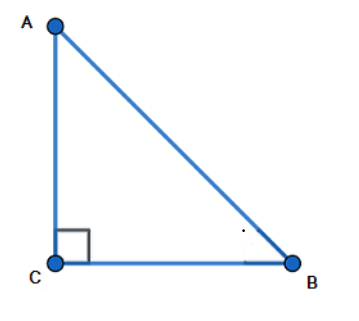Given that $\cos A=\cos B$.

In a right triangle $\Delta ABC$, we know that

$\cos \theta =\dfrac{\text{adjacent side}}{\text{hypotenuse}}$

Here,

$\cos A=\dfrac{AC}{AB}$

And $\cos B=\dfrac{BC}{AB}$

As given $\cos A=\cos B$, we get

$\Rightarrow \dfrac{AC}{AB}=\dfrac{BC}{AB}$

$\Rightarrow AC=AB$

Now, we know that angles opposite to the equal sides are also equal in measure.

Then, we get

$\angle A=\angle B$

Hence proved.

7. Evaluate the following if $\cot \theta =\dfrac{7}{8}$

i) $\dfrac{\left( 1+\sin \theta \right)\left( 1-\sin \theta \right)}{\left( 1+\cos \theta \right)\left( 1-\cos \theta \right)}$

Ans: Let us consider a right angled triangle $\Delta ABC$. We get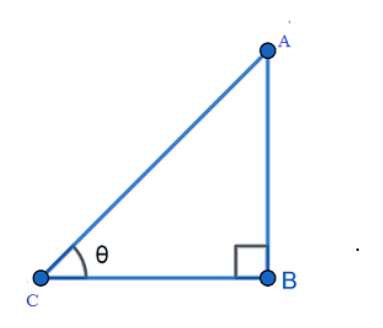Now, in a right triangle we know that $\cot \theta =\dfrac{\text{adjacent side}}{\text{opposite side}}$.

Here, from the figure $\cot \theta =\dfrac{BC}{AB}$ .

We get

$AB=8$ and

$BC=7$

Now, we need to apply the Pythagoras theorem to find the measure of hypotenuse.

In $\Delta ABC$, by Pythagoras theorem ,

${{\left( \text{hypotenuse} \right)}^{2}}={{\left( base \right)}^{2}}+{{\left( perpendicular \right)}^{2}}$

We get

$\Rightarrow {{\left( \text{AC} \right)}^{2}}={{\left( AB \right)}^{2}}+{{\left( BC \right)}^{2}}$

$\Rightarrow {{\left( \text{AC} \right)}^{2}}={{8}^{2}}+{{7}^{2}}$

$\Rightarrow {{\left( \text{AC} \right)}^{2}}=64+49$

$\Rightarrow {{\left( \text{AC} \right)}^{2}}=113$

$\Rightarrow AC=\sqrt{113}$

Now, we know that

$\sin \theta =\dfrac{\text{opposite side}}{\text{hypotenuse}}$

Here, we get

$\sin \theta =\dfrac{AB}{AC}=\dfrac{8}{\sqrt{113}}$ and

$\cos \theta =\dfrac{\text{adjacent side}}{\text{hypotenuse}}$

Here, we get

$\cos \theta =\dfrac{BC}{AC}=\dfrac{7}{\sqrt{113}}$

Now, we have to evaluate

$\dfrac{\left( 1+\sin \theta \right)\left( 1-\sin \theta \right)}{\left( 1+\cos \theta \right)\left( 1-\cos \theta \right)}$

Applying the identity $\left( a+b \right)\left( a-b \right)={{a}^{2}}-{{b}^{2}}$, we get

$\Rightarrow \dfrac{\left( 1+\sin \theta \right)\left( 1-\sin \theta \right)}{\left( 1+\cos \theta \right)\left( 1-\cos \theta \right)}=\dfrac{1-{{\sin }^{2}}\theta }{1-{{\cos }^{2}}\theta }$

Substituting the values, we get

$\Rightarrow \dfrac{\left( 1+\sin \theta \right)\left( 1-\sin \theta \right)}{\left( 1+\cos \theta \right)\left( 1-\cos \theta \right)}=\dfrac{1-{{\left( \dfrac{8}{\sqrt{113}} \right)}^{2}}}{1-{{\left( \dfrac{7}{\sqrt{113}} \right)}^{2}}}$

$\Rightarrow \dfrac{\left( 1+\sin \theta \right)\left( 1-\sin \theta \right)}{\left( 1+\cos \theta \right)\left( 1-\cos \theta \right)}=\dfrac{1-\dfrac{64}{113}}{1-\dfrac{49}{113}}$

$\Rightarrow \dfrac{\left( 1+\sin \theta \right)\left( 1-\sin \theta \right)}{\left( 1+\cos \theta \right)\left( 1-\cos \theta \right)}=\dfrac{\dfrac{113-64}{113}}{\dfrac{113-49}{113}}$

$\Rightarrow \dfrac{\left( 1+\sin \theta \right)\left( 1-\sin \theta \right)}{\left( 1+\cos \theta \right)\left( 1-\cos \theta \right)}=\dfrac{\dfrac{49}{113}}{\dfrac{64}{113}}$

$\therefore \dfrac{\left( 1+\sin \theta \right)\left( 1-\sin \theta \right)}{\left( 1+\cos \theta \right)\left( 1-\cos \theta \right)}=\dfrac{49}{64}$

ii) ${{\cot }^{2}}\theta$

Ans: Given that $\cot \theta =\dfrac{7}{8}$.

Now, ${{\cot }^{2}}\theta ={{\left( \dfrac{7}{8} \right)}^{2}}$

$\therefore {{\cot }^{2}}\theta =\dfrac{49}{64}$

8. If $3\cot A=4$, check whether $\dfrac{1-{{\tan }^{2}}A}{1+{{\tan }^{2}}A}={{\cos }^{2}}A-{{\sin }^{2}}A$ or not.

Ans: Let us consider a right angled triangle $\Delta ABC$. We getGiven that $3\cot A=4$.

We get $\cot A=\dfrac{4}{3}$.

We know that $\cot \theta =\dfrac{\text{adjacent side}}{\text{opposite side}}$.

From the above figure, we get

$\cot A=\dfrac{AB}{BC}$

Therefore, we get

$\Rightarrow BC=3$ and

$\Rightarrow AB=4$

Now, we need to apply the Pythagoras theorem to find the measure of hypotenuse.

In $\Delta ABC$, by Pythagoras theorem ,

${{\left( \text{hypotenuse} \right)}^{2}}={{\left( base \right)}^{2}}+{{\left( perpendicular \right)}^{2}}$

We get

$\Rightarrow {{\left( \text{AC} \right)}^{2}}={{\left( AB \right)}^{2}}+{{\left( BC \right)}^{2}}$

$\Rightarrow A{{C}^{2}}={{4}^{2}}+{{3}^{2}}$

$\Rightarrow A{{C}^{2}}=16+9$

$\Rightarrow A{{C}^{2}}=25$

$\Rightarrow AC=5$

Now, let us consider LHS of the expression $\dfrac{1-{{\tan }^{2}}A}{1+{{\tan }^{2}}A}={{\cos }^{2}}A-{{\sin }^{2}}A$, we get

$LHS=\dfrac{1-{{\tan }^{2}}A}{1+{{\tan }^{2}}A}$

Now, we know that $\tan \theta =\dfrac{\text{opposite side}}{\text{adjacent side}}$

Here, we get

$\tan A=\dfrac{BC}{AB}=\dfrac{3}{4}$

Substitute the value, we get

$\Rightarrow \dfrac{1-{{\tan }^{2}}A}{1+{{\tan }^{2}}A}=\dfrac{1-{{\left( \dfrac{3}{4} \right)}^{2}}}{1+{{\left( \dfrac{3}{4} \right)}^{2}}}$

$\Rightarrow \dfrac{1-{{\tan }^{2}}A}{1+{{\tan }^{2}}A}=\dfrac{1-\dfrac{9}{16}}{1+\dfrac{9}{16}}$

$\Rightarrow \dfrac{1-{{\tan }^{2}}A}{1+{{\tan }^{2}}A}=\dfrac{\dfrac{16-9}{16}}{\dfrac{16+9}{16}}$

$\Rightarrow \dfrac{1-{{\tan }^{2}}A}{1+{{\tan }^{2}}A}=\dfrac{\dfrac{7}{16}}{\dfrac{25}{16}}$

$\Rightarrow \dfrac{1-{{\tan }^{2}}A}{1+{{\tan }^{2}}A}=\dfrac{7}{25}$

Now, let us consider RHS of the expression $\dfrac{1-{{\tan }^{2}}A}{1+{{\tan }^{2}}A}={{\cos }^{2}}A-{{\sin }^{2}}A$, we get

$RHS={{\cos }^{2}}A-{{\sin }^{2}}A$

We know that $\sin \theta =\dfrac{\text{opposite side}}{\text{hypotenuse}}$ and $\cos \theta =\dfrac{\text{adjacent side}}{\text{hypotenuse}}$.

Here, we get

$\sin A=\dfrac{BC}{AC}=\dfrac{3}{5}$

And $\cos A=\dfrac{AB}{AC}=\dfrac{4}{5}$

Substitute the values, we get

$\Rightarrow {{\cos }^{2}}A-{{\sin }^{2}}A={{\left( \dfrac{4}{5} \right)}^{2}}-{{\left( \dfrac{3}{5} \right)}^{2}}$

$\Rightarrow {{\cos }^{2}}A-{{\sin }^{2}}A=\dfrac{16}{25}-\dfrac{9}{25}$

$\Rightarrow {{\cos }^{2}}A-{{\sin }^{2}}A=\dfrac{7}{25}$

Hence, we get LHS=RHS

$\therefore \dfrac{1-{{\tan }^{2}}A}{1+{{\tan }^{2}}A}={{\cos }^{2}}A-{{\sin }^{2}}A$.

9. In $ABC$, right angled at $B$. If $\tan A=\dfrac{1}{\sqrt{3}}$, find the value of

i) $\sin A\cos C+\cos A\sin C$

Ans: Let us consider a right angled triangle $\Delta ABC$. We getGiven that $\tan A=\dfrac{1}{\sqrt{3}}$.

In a right triangle, we know that $\tan \theta =\dfrac{\text{opposite side}}{\text{adjacent side}}$

Here, from the figure we get

$\tan A=\dfrac{BC}{AB}=\dfrac{1}{\sqrt{3}}$

We get $BC=1$ and $AB=\sqrt{3}$ .

Now, we need to apply the Pythagoras theorem to find the measure of hypotenuse.

In $\Delta ABC$, by Pythagoras theorem ,

${{\left( \text{hypotenuse} \right)}^{2}}={{\left( base \right)}^{2}}+{{\left( perpendicular \right)}^{2}}$

We get

$\Rightarrow {{\left( \text{AC} \right)}^{2}}={{\left( AB \right)}^{2}}+{{\left( BC \right)}^{2}}$

$\Rightarrow A{{C}^{2}}={{\left( \sqrt{3} \right)}^{2}}+{{1}^{2}}$

$\Rightarrow A{{C}^{2}}=3+1$

$\Rightarrow A{{C}^{2}}=4$

$\Rightarrow AC=2$

We know that $\sin \theta =\dfrac{\text{opposite side}}{\text{hypotenuse}}$ and $\cos \theta =\dfrac{\text{adjacent side}}{\text{hypotenuse}}$.

Here, we get

$\sin A=\dfrac{BC}{AC}=\dfrac{1}{2}$  and $\sin C=\dfrac{AB}{AC}=\dfrac{\sqrt{3}}{2}$

And $\cos A=\dfrac{AB}{AC}=\dfrac{\sqrt{3}}{2}$and $\cos C=\dfrac{BC}{AC}=\dfrac{1}{2}$

Now, we have to find the value of the expression $\sin A\cos C+\cos A\sin C$.

Substituting the values we get

$\Rightarrow \sin A\cos C+\cos A\sin C=\dfrac{1}{2}\times \dfrac{1}{2}+\dfrac{\sqrt{3}}{2}\times \dfrac{\sqrt{3}}{2}$

$\Rightarrow \sin A\cos C+\cos A\sin C=\dfrac{1}{4}+\dfrac{3}{4}$

$\Rightarrow \sin A\cos C+\cos A\sin C=\dfrac{4}{4}$

$\therefore \sin A\cos C+\cos A\sin C=1$

ii) $\cos A\cos C-\sin A\sin C$

Ans: Let us consider a right angled triangle $\Delta ABC$. We getGiven that $\tan A=\dfrac{1}{\sqrt{3}}$.

In a right triangle, we know that $\tan \theta =\dfrac{\text{opposite side}}{\text{adjacent side}}$

Here, from the figure we get

$\tan A=\dfrac{BC}{AB}=\dfrac{1}{\sqrt{3}}$

We get $BC=1$ and $AB=\sqrt{3}$ .

Now, we need to apply the Pythagoras theorem to find the measure of hypotenuse.

In $\Delta ABC$, by Pythagoras theorem ,

${{\left( \text{hypotenuse} \right)}^{2}}={{\left( base \right)}^{2}}+{{\left( perpendicular \right)}^{2}}$

We get

$\Rightarrow {{\left( \text{AC} \right)}^{2}}={{\left( AB \right)}^{2}}+{{\left( BC \right)}^{2}}$

$\Rightarrow A{{C}^{2}}={{\left( \sqrt{3} \right)}^{2}}+{{1}^{2}}$

$\Rightarrow A{{C}^{2}}=3+1$

$\Rightarrow A{{C}^{2}}=4$

$\Rightarrow AC=2$

We know that $\sin \theta =\dfrac{\text{opposite side}}{\text{hypotenuse}}$ and $\cos \theta =\dfrac{\text{adjacent side}}{\text{hypotenuse}}$.

Here, we get

$\sin A=\dfrac{BC}{AC}=\dfrac{1}{2}$  and $\sin C=\dfrac{AB}{AC}=\dfrac{\sqrt{3}}{2}$

And $\cos A=\dfrac{AB}{AC}=\dfrac{\sqrt{3}}{2}$and $\cos C=\dfrac{BC}{AC}=\dfrac{1}{2}$

Now, we have to find the value of the expression $\cos A\cos C-\sin A\sin C$.

Substituting the values we get

$\Rightarrow \cos A\cos C-\sin A\sin C=\dfrac{\sqrt{3}}{2}\times \dfrac{1}{2}-\dfrac{1}{2}\times \dfrac{\sqrt{3}}{2}$

$\Rightarrow \cos A\cos C-\sin A\sin C=\dfrac{\sqrt{3}}{4}-\dfrac{\sqrt{3}}{4}$

$\therefore \Rightarrow \cos A\cos C-\sin A\sin C=0$

10. In $\Delta PQR$, right angled at $Q$, $PR+QR=25\text{ cm}$ and $PQ=5\text{ cm}$. Determine the values of $\sin P,\cos P$ and $\tan P$.

Ans: Let us consider a right angled triangle $\Delta PQR$, we get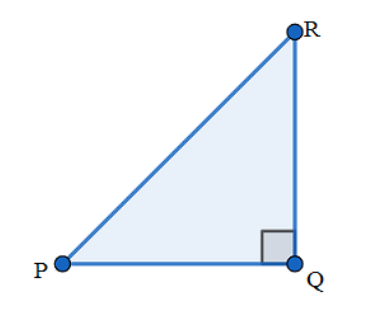Given that $PR+QR=25\text{ cm}$ and $PQ=5\text{ cm}$.

Let $QR=25-PR$

Now, applying the Pythagoras theorem in $\Delta PQR$, we get

${{\left( \text{hypotenuse} \right)}^{2}}={{\left( base \right)}^{2}}+{{\left( perpendicular \right)}^{2}}$

We get

$\Rightarrow {{\left( PR \right)}^{2}}={{\left( PQ \right)}^{2}}+{{\left( QR \right)}^{2}}$

$\Rightarrow P{{R}^{2}}={{5}^{2}}+{{\left( 25-PR \right)}^{2}}$

$\Rightarrow P{{R}^{2}}=25+{{25}^{2}}+P{{R}^{2}}-50PR$

$\Rightarrow P{{R}^{2}}=P{{R}^{2}}+25+625-50PR$

$\Rightarrow 50PR=650$

$\Rightarrow PR=13\text{ cm}$

Therefore,

$QR=25-13$

$\Rightarrow QR=12\text{ cm}$

Now, we know that in right triangle,

$\sin \theta =\dfrac{\text{opposite side}}{\text{hypotenuse}}$, $\cos \theta =\dfrac{\text{adjacent side}}{\text{hypotenuse}}$ and $\tan \theta =\dfrac{\text{opposite side}}{\text{adjacent side}}$.

Here, we get

$\sin P=\dfrac{QR}{PR}$

$\therefore \sin P=\dfrac{12}{13}$

$\cos P=\dfrac{PQ}{PR}$

$\therefore \cos P=\dfrac{5}{13}$

$\tan P=\dfrac{QR}{PQ}$

$\therefore \tan P=\dfrac{12}{5}$

10. State whether the following are true or false. Justify your answer.

i) The value of $\tan A$ is always less than $1$.

Ans: The given statement is false. The value of $\tan A$ depends on the length of sides of a right triangle and sides of a triangle may have any measure.

ii) For some value of angle $A$, $\sec A=\dfrac{12}{5}$.

Ans: We know that in the right triangle $\sec A=\dfrac{\text{hypotenuse}}{\text{adjacent side of }\angle \text{A}}$ .

We know that in the right triangle the hypotenuse is the largest side.

Therefore, the value of $\sec A$ must be greater than $1$.

In the given statement $\sec A=\dfrac{12}{5}$, which is greater than $1$.

Therefore, the given statement is true.

iii) $\cos A$ is the abbreviation used for the cosecant of angle $A$.

Ans: The given statement is false because $\cos A$ is the abbreviation used for the cosine of angle $A$. Abbreviation used for the cosecant of angle $A$ is $\operatorname{cosec}A$.

iv) $\cot A$ is the product of $\cot$ and $A$.

Ans: $\cot A$ is the abbreviation used for the cotangent of angle $A$. Hence the given statement is false.

v) For some angle $\theta$, $\sin \theta =\dfrac{4}{3}$.

Ans: We know that in the right triangle $\sin \theta =\dfrac{\text{opposite side}}{\text{hypotenuse}}$ .

We know that in the right triangle the hypotenuse is the largest side.

Therefore, the value of $\sin \theta$ must be less than $1$.

In the given statement $\sin \theta =\dfrac{4}{3}$, which is greater than $1$.

Therefore, the given statement is false.

## NCERT Solutions for Class 10 Maths Chapter 8 Introduction to Trigonometry Exercise 8.1

### Exercise 8.1 Class 10 Solutions Chapter 8 Trigonometry- Free PDF Download

As Vedantu's main ambition is student's success, Vedantu provides these notes for free download on Vedantu. Class 10 Maths Chapter 8 exercise 8.1 Solutions can be downloaded in the form of PDF for free, which can be used for any future references by printing a hardcopy of the same.

The reference material compiled by the Experts of Vedantu is up to date with the NCERT syllabus. Maths Chapter 8 exercise 8.1 Class 10 Solutions strengthens the fundamentals of the students. Solutions to all the questions are sequential, making it easy for the students to navigate through the PDF Download. Pictorial representation of all the answers makes it highly efficient as they are interactive and conceptual understanding improves.

### Class 10th Maths Chapter 8 Exercise 8.1

Maths NCERT class 10 ex 8.1 introduces the students to the fundamentals of trigonometry. This exercise majorly concentrates on the basics of trigonometry, which is essential for the upcoming classes. The branch of mathematics in which the heights and angles of a right triangle can be correlated is trigonometry. The word 'trigonometry' is derived from the Greek words 'tri' (meaning three), 'gon' (meaning sides), and 'metron' (meaning measure) justifying its relationship with the three-sided polygon triangle. Class 10th Maths Chapter 8 exercise 8.1 focuses on the trigonometric ratios of the angle. The angles discussed here are predominantly acute angles, for simplicity, though it can be extended to obtuse angles.

Trigonometry has a lot of real-life applications- it is used in various industries, and even simple distance height problems can be solved with trigonometry. Those types of real-life problem-based questions will also be encountered by the student later in Maths Chapter 8 exercise 8.1.

There are six trigonometric ratios of angle, namely sin, cos, tan, cosec, sec, cot. Of which cosec, sec, and cot are the inverse of sin cos and tan about which you would learn more in detail in Class 10 Maths Chapter 8 exercise 8.1.

In the right triangle, the side opposite to the right angle (i.e., 90 degrees angle) is called the hypotenuse. Let us consider a non-right acute angle of the triangle, say A, and the trigonometric ratios are defined based on the adjacent side of the angle and the opposite side of the angle. The trigonometric ratios are represented as sin A meaning sin of angle A.

Class 10 Maths ex 8.1 also includes the relationship between the trigonometric ratios of the angle. This is well addressed in the Exercise 8.1 class 10 solutions. Referring to these notes for a better understanding of the relationship between the trigonometric ratios of the angle. Students can also apply it in the right place for easy results. Adopting these techniques can reduce the time consumed to solve a problem significantly, helping students manage time during the examination.

It is observed in the problems of Class 10 Trigonometry Exercise 8.1 that the values of the trigonometric ratios of an angle do not vary with the lengths of the sides of the triangle if the angle remains the same. In Maths class 10 ex 8.1 solutions, the angles are represented by the Greek letter theta.

Chapter 8 Maths Class 10 Exercise 8.1 deals with the types of problems like determining the trigonometric ratios given the sides of the triangle, finding the other trigonometric ratios given one of them by constructing a triangle of the respective ratio. Like above-mentioned usage of relations between the trigonometric ratio of the angles can ease the process of solving the problem. Also, operations with the trigonometric ratio might be asked to perform and draw the result values.

Exercise 8.1 class 10 solutions can be extremely useful when it comes to learning the concepts. The formulas and identities associated with the trigonometric ratios are at their fingertips after referring to these notes and practicing the questions from Exercise 8.1 class 10 solutions Chapter 8 Trigonometry.

### NCERT Solutions for Class 10 Maths Chapter 8 All Other Exercises

 Chapter 8 - Introduction to Trigonometry Exercises in PDF Format Exercise 8.2 4 Questions & Solutions (2 Short Answers, 2 Long Answers) Exercise 8.3 7 Questions & Solutions (5 Short Answers, 2 Long Answers) Exercise 8.4 5 Questions & Solutions (3 Short Answers, 2 Long Answers)

## FAQs on NCERT Solutions for Class 10 Maths Chapter 8 - Exercise

1. What are the formulas to calculate the trigonometric ratio of the angles?

Consider a right-angled triangle of an acute angle A

sin A = Side opposite to angle A / Hypotenuse

cos A = Side adjacent to angle A / Hypotenuse

tan A = Side opposite to angle A / Side adjacent to angle A

cosec A = Hypotenuse / Side opposite to angle A

sec A = Hypotenuse / Side adjacent to angle A

cot A = Side adjacent to angle A / Side opposite to angle A

are the formulas for calculating the trigonometric ratio of the angles.

2. Why is the Class 10 Trigonometry Exercise 8.1 important?

Class 10 Trigonometry Exercise 8.1 covers the fundamentals of the vast branch of mathematics, namely trigonometry. This concept is crucial for understanding the concepts of the student's higher education irrespective of the stream they choose. These concepts are explained and are broken down to their simplest form in Exercise 8.1 Class 10 Maths Ncert solutions. Learning these concepts by heart helps the students score significantly high in their examinations. As the CBSE Board question paper gives a pretty high weightage to this chapter, it's easy to score well once the concepts are well-learned.

3. How Vedantu NCERT solutions help in examination times?

NCERT solutions of Vedantu help students to frame mind maps of the type of problems that they may encounter in their examination. The mentors of Vedantu train the students to face the exams with confidence. Easier learning of concepts lessens the preparation time, which in turn reflects in the student's Examination tactics. Knowing their strengths and weaknesses helps the student to plan their methodology to attend to the questions with the time constraint in mind.

4. What are the topics discussed in exercise 8.1 ?

Chapter 8 in Class 10 Maths is trigonometry. Trigonometry studies ratios and angles of triangles. Exercise 8.1 of the textbook focuses on the basics of trigonometry. It introduces them to questions with acute angles. It forms a basis for topics that would be discussed in further exercises and classes. It is a fairly easy exercise. The students can find step by step solutions in the solutions PDF provided by Vedantu online on the website.

5. How many questions does Exercise 8.1 have?

Exercise 8.1 is the first exercise in Chapter 8 of Class 10 Maths. The chapter introduces the basics and fundamentals of trigonometry. It is an important topic that is studied in classes ahead as well. There are 11 questions in the exercise discussing ratios and angels. The solutions for all questions can be found in the solution pdf for the reference of students. They are explained in an easy language for the students to understand better.

6. Why is Chapter 8 of Class 10 Maths important?

Chapter 8 of Class 10 is called trigonometry. It introduces students to the basics of the topic and aims at helping them form a strong base. This topic is important as it is discussed in further classes and in colleges as well depending on the subjects chosen by the student. It is a topic that is discussed in daily life as well. The topic requires the concentration of the students and regular practice. The students can score highly in it if they practice enough.

7. How many exercises are there in chapter 8 Trigonometry?

There are a total of four exercises in chapter 8- Exercise 8.1, 8.2, 8.3 and 8.4. The exercises cover all the topics and formulas discussed throughout the chapter. Each exercise covers a few particular topics and formulas. The exercises aim at making the student comfortable and well-versed with the topic. The students can refer to the solutions PDF for answers and extra questions for Trigonometry. Answers to the NCERT textbook exercises are explained step by step in easy language.

8. How should I prepare chapter 8 for the final exam?

Trigonometry requires the students to be thorough and regular with the topic and all the questions in the NCERT textbook. They should also practice extra questions from the PDF to form a stronger base on the subject. Students should regularly revise what they are being taught in school. They should also write down all the formulas so that it is easy for them to revise and they can retain them better. If they understand the topic they will be able to score good marks in the subject.